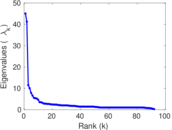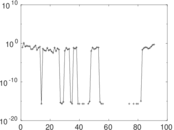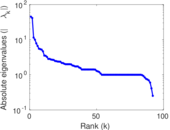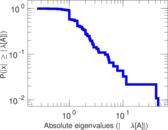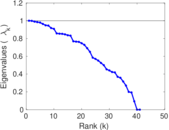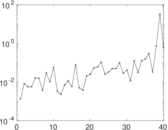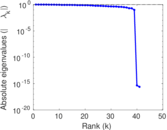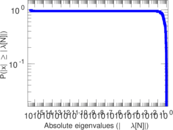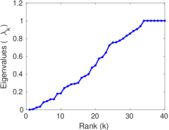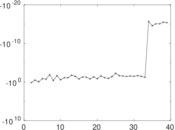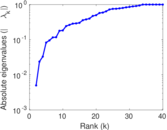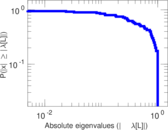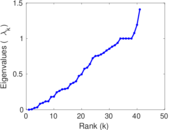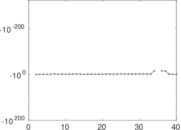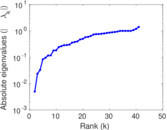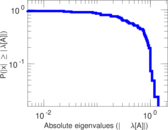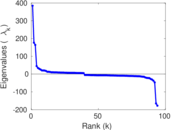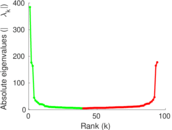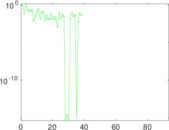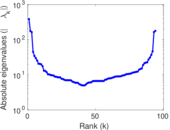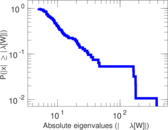# Wikiquote edits (ast)

This is the bipartite edit network of the Asturian Wikiquote. It contains users and pages from the Asturian Wikiquote, connected by edit events. Each edge represents an edit. The dataset includes the timestamp of each edit.

 Code `qast` Internal name `edit-astwikiquote` Name Wikiquote edits (ast) Data source http://dumps.wikimedia.org/ AvailabilityDataset is available for download Consistency checkDataset passed all tests Category Authorship network Dataset timestamp 2017-10-20 Node meaning User, article Edge meaning Edit Network formatBipartite, undirected Edge typeUnweighted, multiple edges Temporal dataEdges are annotated with timestamps

## Statistics

 Size n = 394 Left size n1 = 94 Right size n2 = 300 Volume m = 637 Unique edge count m̿ = 381 Wedge count s = 5,532 Claw count z = 130,800 Cross count x = 2,731,079 Square count q = 122 4-Tour count T4 = 24,010 Maximum degree dmax = 164 Maximum left degree d1max = 164 Maximum right degree d2max = 41 Average degree d = 3.233 50 Average left degree d1 = 6.776 60 Average right degree d2 = 2.123 33 Fill p = 0.013 510 6 Average edge multiplicity m̃ = 1.671 92 Size of LCC N = 248 Diameter δ = 18 50-Percentile effective diameter δ0.5 = 5.474 54 90-Percentile effective diameter δ0.9 = 9.619 49 Median distance δM = 6 Mean distance δm = 6.029 83 Gini coefficient G = 0.668 043 Balanced inequality ratio P = 0.233 124 Left balanced inequality ratio P1 = 0.207 221 Right balanced inequality ratio P2 = 0.315 542 Relative edge distribution entropy Her = 0.881 121 Power law exponent γ = 4.436 39 Tail power law exponent γt = 2.491 00 Tail power law exponent with p γ3 = 2.491 00 p-value p = 0.987 000 Left tail power law exponent with p γ3,1 = 2.251 00 Left p-value p1 = 0.917 000 Right tail power law exponent with p γ3,2 = 3.051 00 Right p-value p2 = 0.249 000 Degree assortativity ρ = −0.264 050 Degree assortativity p-value pρ = 1.692 98 × 10−7 Spectral norm α = 45.056 9 Algebraic connectivity a = 0.004 979 90 Spectral separation |λ1[A] / λ2[A]| = 1.092 47 Controllability C = 207 Relative controllability Cr = 0.529 412

## Plots

### Fruchterman–Reingold graph drawing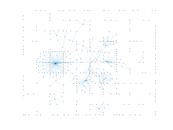### Degree distribution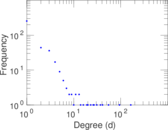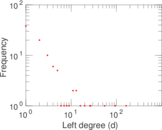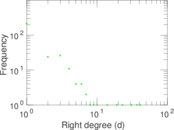### Cumulative degree distribution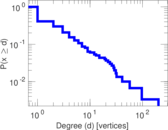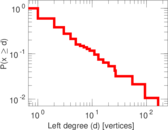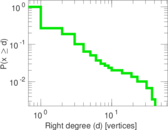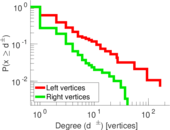### Lorenz curve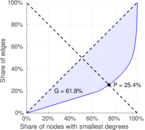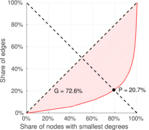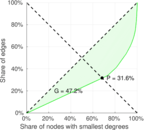### Spectral distribution of the adjacency matrix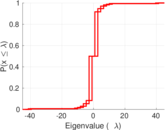### Spectral distribution of the normalized adjacency matrix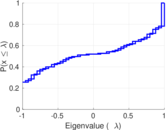### Spectral distribution of the Laplacian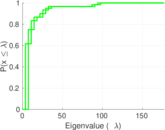### Spectral graph drawing based on the adjacency matrix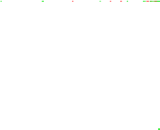### Spectral graph drawing based on the Laplacian### Spectral graph drawing based on the normalized adjacency matrix### Degree assortativity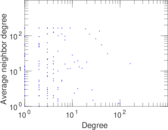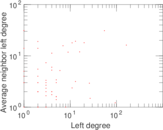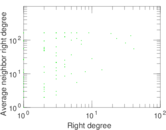### Zipf plot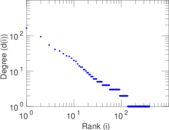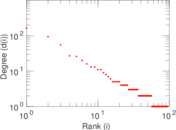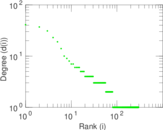### Hop distribution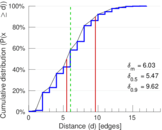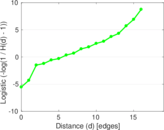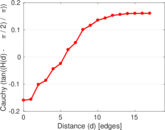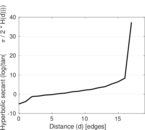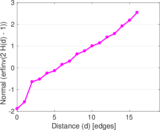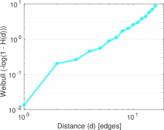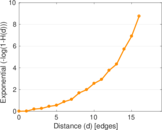### Double Laplacian graph drawing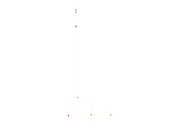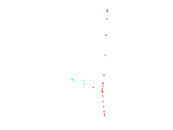### Delaunay graph drawing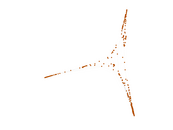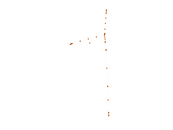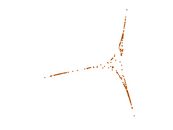### Edge weight/multiplicity distribution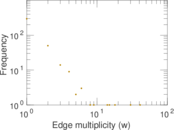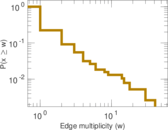### Temporal distribution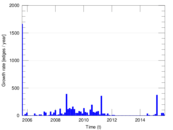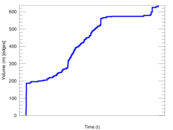### Temporal hop distribution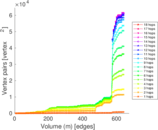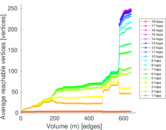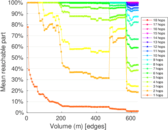### Diameter/density evolution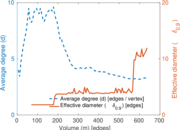### Matrix decompositions plots